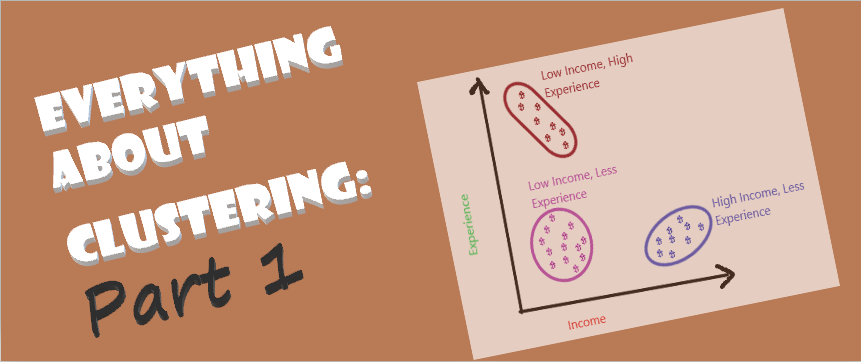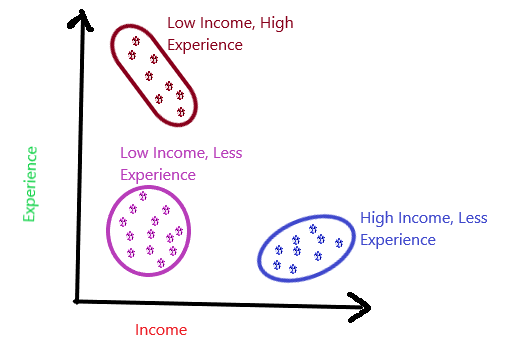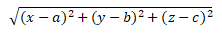New Delhi, India

# Introduction To Clustering# Introduction

Hi Everyone! I am starting with the clustering series from today. Let’s learn what it is in general in this article. This post will cover the its theory.

## What is Clustering?

Clustering is defined as the organizing or bringing together elements which are of similar type or nature.

For example, if I talk about dogs, then we all know that there are many breeds of dogs. I can group the dogs into different breeds on the basis of different features and nature each of them are having and these groups are what we call as the clusters. That is, in each cluster, the dogs are of same type or are homogeneous in nature. We bring similar dogs in one cluster and dissimilar dogs are kept in different cluster.

For better understanding, let’s take another example. The following graph shows income on x-axis and experience level on y-axis. Here, we have formed three clusters. Thus, we have grouped the points which are having high income but less experience in one cluster, low income and less experience in another cluster and low income and high experience in the third cluster.## The Objective Behind Clustering

 Intra-clustering Distance Inter-clustering Distance This is the sum total of the distances between the objects of the same clusters. This the distance between the objects of different clusters. This distance should be minimized. This distance should be maximized.

## Clustering and the Distances

To understand more about how this is done, look at the following figure. It shows three points with their respective co-ordinates on the graph:

point 1: (1000, 200)

point 2: (2000, 800)

point 3: (6000, 500)Now, there are many ways of calculating the distances between two points. They are:

• Euclidean distance
• Manhattan distance
• Mahalanobis distance

Out of all three, Euclidean distance is used the most. So, let’s talk about it.

The Euclidean distance between two points (a, b, c) and (x, y, z) is calculated using the following formula:Therefore, distance between point 1 and point 2 is 1166, that of point 2 and point 3 is 4011 and of point 1 and point 3 is 5009. Out of all three, the distance between point 1 and point 2 is the least. So, we put them in the same cluster, cluster A and point 3 is in cluster B. This is how we do the clustering using Euclidean distance.

## The Concept of Scaling

In the above example, you must have noticed one thing. That is, I have taken the dimensions on different scales. That is, y-axis is increasing in 1000s and x-axis is increasing in hundreds. Thus, if you calculate the above distances manually, you will notice that the difference between the y coordinates is magnified to a greater extent than that of x coordinates. This poses a huge problem if one variable has the values in tens of numbers and another has in 1000s. This actually results in incorrect clustering as the difference between one of the coordinates outweighs that of another.

To combat this, we use scaling. That is, we manipulate the variables so that they come in same scale. We use many techniques to do this: standardization, normalization to name a few.

In standardization, each observation in a particular variable is subtracted by the mean of the variable and the resulting difference is divided by standard deviation. Thus, each observation is scaled down to ones scale.

In normalization, each observation is subtracted by the minimum value of the variable. The difference is then divided by the range of the variable. (Range = maximum value – minimum value of the variable).

When this is done, you are ready to apply the algorithms of clustering.

## Types of Clustering

There are many methods of clustering. They are:

(i) Hierarchical clustering which works on the principle that the point which is closer to a base point will perform in a more similar manner to the base point than the point which is farther away from the base point. It can further be divided into Agglomerative(bottom-up) and Divisive(top-down). In Divisive clustering, approach is to break down big cluster into smaller clusters. In Agglomerative clustering, the approach is to start with separate smaller clusters and combine them into one cluster.

(ii) Partitional clustering such as K-means clustering which goes on determining all clusters at once.

(iii) Grid-based clustering

So, guys this was all about clustering in general. In the upcoming articles, we will learn about its methods in greater details. Stay tuned to this series!

## One thought on “Introduction To Clustering”

This site uses Akismet to reduce spam. Learn how your comment data is processed.